• 估计逻辑回归模型时经常遇到的问题是似然最大化算法无法收敛。 尽管在对逻辑回归的参数的最大似然估计进行偏差校正时非常流行并且建立得很好，但是对最大似然方法的行为和性质的研究较少。 本文的主要目的是利用归约...
• ## 逻辑回归与最大似然估计推导

万次阅读 多人点赞 2018-08-01 16:42:31
3. 极大似然估计 4. 利用梯度下降法求解参数w 4.1 三种梯度下降方法的选择 5.逻辑回归优缺点： 参考资料： 逻辑回归（对数几率回归） 逻辑回归是一种分类算法，不是回归算法，因为它用了和回归类似的思想来...
目录
逻辑回归（对数几率回归）
1.广义线性模型
2.逻辑回归的假设
3. 逻辑回归的代价函数
为什么LR中使用交叉熵损失函数而不使用MSE损失函数？
3. 极大似然估计
4. 利用梯度下降法求解参数w
4.1 三种梯度下降方法的选择
5.逻辑回归优缺点：
参考资料：

逻辑回归（对数几率回归）
逻辑回归是一种分类算法，不是回归算法，因为它用了和回归类似的思想来解决了分类问题。
一句话总结逻辑回归：“逻辑回归假设数据服从伯努利分布，通过极大似然函数的方法，运用梯度下降来求解参数，来达到将数据二分类的目的”。
1.广义线性模型
我们先来看看线性回归模型：

但是假设我们认为实例所对应的输出标记是在指数尺度上变化，那么就可以将输出标记的对数作为线性模型逼近的目标：

这就是“对数线性回归”，它实际上是试图让逼近y，其是形式上是线性回归，实际上是在求输入空间到输出空间的非线性函数映射。这里的对数函数起到了将线性回归模型的预测值与真实标记联系起来的作用。
更一般地，考虑单调可微函数，另：

这样得到的模型称为“广义线性模型”，其中函数称为“联系函数”。显然，对数线性回归是广义线性模型在时的特例。
如上讨论了如何使用线性模型来进行回归学习，但是如果要做的是分类任务该怎么办？下面介绍如何由“广义线性模型”引出逻辑回归模型。
我们只需要找到一个联系函数，将分类任务的真实标记y与线性回归模型的预测值联系起来。
考虑二分类问题，其输出标记，而线性回归模型的预测值是实数值，于是我们需要将实数值z转换为0/1值，可以使用单位阶跃函数：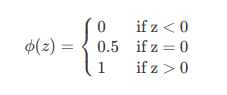但是该函数不连续，因此不能作为联系函数g，所以找到了一个能在一定程度上近似单位阶跃函数的“替代函数”，单调可微的对数几率函数（Logistic function），它能够将线性回归模型的预测值转化为分类任务对应的概率:

两者的图像如下图所示：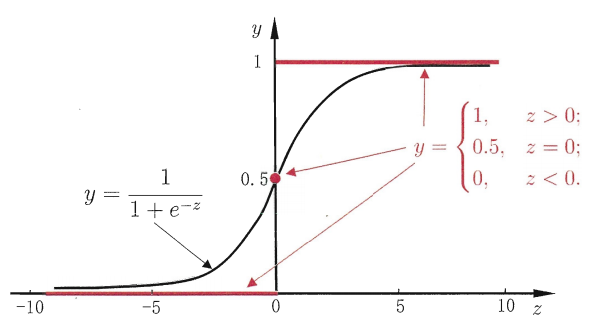图1.单位阶跃函数与对数几率函数

对数几率其实是一种“sigmoid”函数，它将z值转化为一个接近0或1的y值：

若将y视为样本x作为正例的可能性，则1-y是其反例的可能性，两者的比值称为“几率”，反映了x作为正例的相对可能性，对几率取对数则得到，可以看出，上式其实是在用线性回归模型的预测结果去逼近真实标记的对数几率。所以该模型也被称作“对数几率回归”。

由于sigmoid函数的取值在[0,1]之间，所以可以将其视为类1的后验概率估计，所以我们把sigmoid函数计算得到的值大于等于0.5的归为类别1，小于0.5的归为类别0。

面经问题：
1. 为什么要使用sigmoid函数作为假设？
因为线性回归模型的预测值为实数，而样本的类标记为（0,1），我们需要将分类任务的真实标记y与线性回归模型的预测值联系起来，也就是找到广义线性模型中的联系函数。如果选择单位阶跃函数的话，它是不连续的不可微。而如果选择sigmoid函数，它是连续的，而且能够将z转化为一个接近0或1的值。
2．使用sigmoid函数背后的原理是什么？

2.逻辑回归的假设
任何的模型都是有自己的假设的，在这个假设下模型才是试用的。
逻辑回归的第一个假设是：假设数据服从伯努利分布。第二个假设为假设模型的输出值是样本为正例的概率。
所以整个模型可以描述为：
其中=（w;b）为向量形式。
3. 逻辑回归的代价函数
为什么LR中使用交叉熵损失函数而不使用MSE损失函数？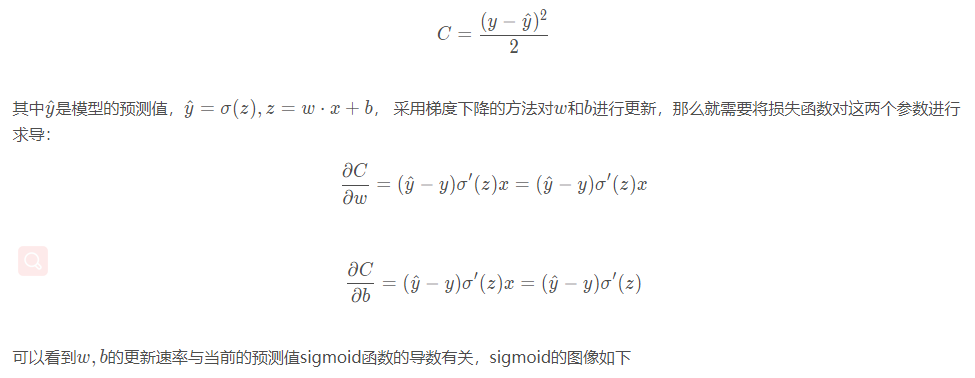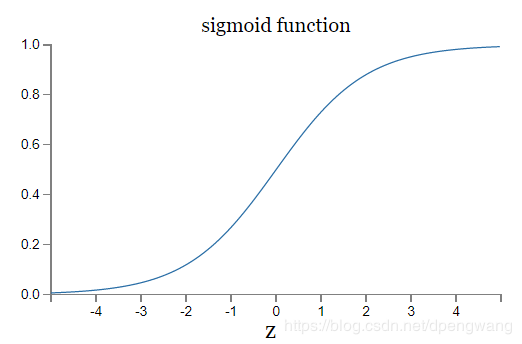所以，如果当前模型的输出接近0或者1时，σ′(z)就会非常小，接近0，使得求得的梯度很小，损失函数收敛的很慢．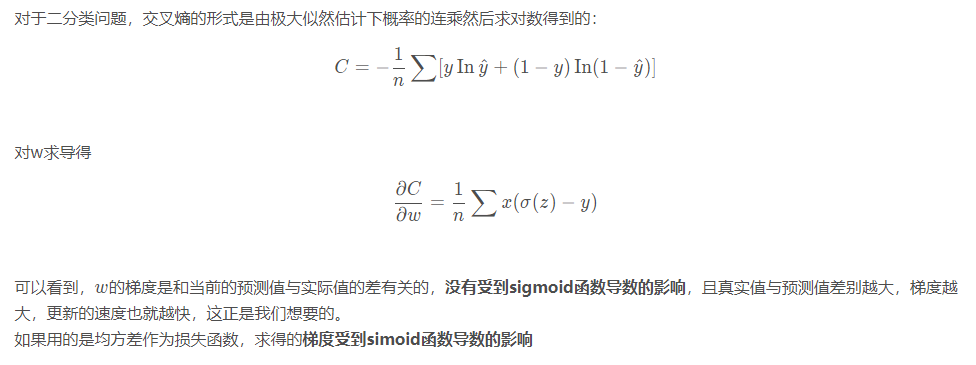（接下来就是根据给定的训练集，把参数w求出来。要找到w，首先要先定义代价函数（目标函数）。首先想到的就是模仿线性回归的做法，利用误差平方和来当做代价函数：

将带入的话，会发现这是一个非凸函数，这就意味着代价函数有着许多的局部最小值，不利于求解。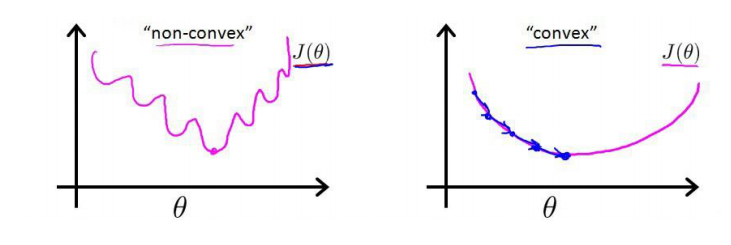而最大似然作为逻辑回归模型的损失函数，很容易得到参数的最优解（凸函数）。所以说选取的标准要容易测量，这就是逻辑回归损失函数为什么使用最大似然而不用最小二乘的原因。
（为什么MSE是非凸函数，有人能解答一下嘛））
3. 极大似然估计
逻辑回归与极大似然估计的关系：
最大似然估计就是通过已知结果去反推最大概率导致该结果的参数。极大似然估计是概率论在统计学中的应用，它提供了一种给定观察数据来评估模型参数的方法，即 “模型已定，参数未知”，通过若干次试验，观察其结果，利用实验结果得到某个参数值能够使样本出现的概率为最大，则称为极大似然估计。逻辑回归是一种监督式学习，是有训练标签的，就是有已知结果的，从这个已知结果入手，去推导能获得最大概率的结果参数，只要我们得出了这个参数，那我们的模型就自然可以很准确的预测未知的数据了。
之前提到了可以视为类1的后验概率，所以有：

将上面两式写为一般形式：

接下来使用极大似然估计来根据给定的训练集估计出参数w：

为了简化运算，我们对上述等式两边取一个对数：

现在要求使得最大的，在前面加一个负号就变为最小化负对数似然函数：

如此就得到了代价函数。让我们更好地理解这个代价函数：

等价于：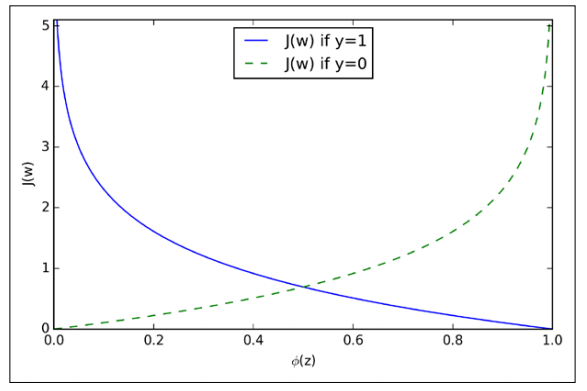可以看出，如果样本的类别为1，估计值越接近1付出的代价越小，反之越大。
同理，如果样本的值为0的话，估计值越接近于0付出的代价越小，反之越大。
4. 利用梯度下降法求解参数w
首先解释一些问什么梯度的负方向就是代价函数下降最快的方向，借助于泰勒展开：

和均为向量，那么两者的内积就等于：

当时，也就是在的负方向时，取得最小值，也就是下降的最快方向了。
梯度下降：

为学习率，用来控制步长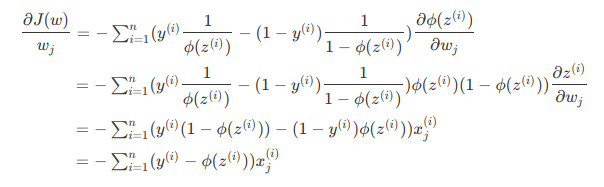所以，在使用梯度下降法更新权重时，只要根据下式即可：

（代表第j列特征，代表第j个特征对应的参数）
当样本量极大的时候，每次更新权重都需要遍历整个数据集，会非常耗时，这时可以采用随机梯度下降法：

每次仅用一个样本点来更新回归系数，这种方法被称作随机梯度上升法。（由于可以在新样本到来时对分类器进行增量式更新，因此随机梯度算法是一个在线学习算法。）它与梯度上升算法效果相当，但占用更少的资源。
4.1 三种梯度下降方法的选择
在实际应用时根据样本量的大小选择不同的梯度更新方法。
5.逻辑回归优缺点
优点：
1.直接对分类可能性进行建模，无需实现假设数据分布，这样就避免了假设分布不准确所带来的问题（周志华.机器学习）
（其实很多机器学习模型本身都是对数据分布有一定的假设的，在这个假设前提之下去进行理论研究有助于我们关注主要矛盾，忽律次要矛盾。但是在工程当中，很多时候我们对数据的分布其实是不了解的，贸然对数据进行假设容易造成模型无法无法拟合真实的分布。）
2.形式简单，模型的可解释性非常好，特征的权重可以看到不同的特征对最后结果的影响。
2.除了类别，还能得到近似概率预测，这对许多需利用概率辅助决策的任务很有用。
3.对率函数是任意阶可导的凸函数，有很好的数学性质。
缺点：
1.准确率不是很高，因为形势非常的简单，很难去拟合数据的真实分布？
2.本身无法筛选特征。
参考资料
1.周志华.机器学习
2.逻辑回归(logistic regression)的本质——极大似然估计
3.逻辑回归的常见面试点总结
4.https://blog.csdn.net/dpengwang/article/details/96597606


展开全文• 极大似然估计回归 极大似然估计 先简单说下似然(likelihood)和概率(probability)的区别，两者都是对可能性的表示。概率是在给定了一定参数值后，表示了一件事物发生的可能性；而似然则反其道而行之，是在给定...
极大似然估计与回归
极大似然估计
先简单说下似然(likelihood)和概率(probability)的区别，两者都是对可能性的表示。概率是在给定了一定参数值后，表示了一件事物发生的可能性；而似然则反其道而行之，是在给定了一系列结果后，表示了某一组参数值的可能性。那么最大似然估计的思想，就是在给定了一组结果后哪一组参数的可能性最大；反过来说，就是使用这样一组参数，出现给定结果的可能性最大。即条件概率

P(X|Θ)=L(Θ|X)
$P(X|\Theta) = \mathcal{L}(\Theta|X)$。
根据机器学习的目的，我们就是在找寻一组参数，这组参数可以让机器取代人工进行分类、拟合等功能，从定义的角度来看，极大似然估计似乎正是我们所需要的。下面我们就用极大似然估计的方法来重新学习下线性回归和逻辑回归（LR）。
线性回归
最小二乘法
想必大家已经对线性回归足够的了解，再简单回顾一下，给定一组样本

X
$X$ 和结果 Y$Y$，希望得到一组参数

Θ
$\Theta$ 从而能够尽可能使得

Yi
$Y_{i}$ 和

XTiΘ
$X_{i}^T\Theta$ 相近 。假设

Yi^=XTiΘ
$\hat{Y_{i}} = X_{i}^T\Theta$，那么对于样本

Xi
$X_{i}$ 来说，其估计的误差就是

|Yi−Yi^|
$|Y_{i} - \hat{Y_{i}}|$。那么我们想要得到一组最好的参数

Θ
$\Theta$，就代表我们要尽可能缩小所有样本的误差之和，这就是最小二乘法的本质。
根据上面的回顾，我们很容易得到常用的代价函数：

J(Θ)=12m∑i=0m(Yi−Yi^)2
$\mathcal{J}(\Theta) = \frac{1}{2m}\sum\limits_{i=0}^{m}(Y_{i} - \hat{Y_{i}})^2$

而我们接下来要做的就是最小化这个代价函数从而能够找到一组参数

Θ
$\Theta$ 使得总误差最小。
极大似然法
那么我们现在就从极大似然估计的角度来看一下线性回归的本质。现在我们假设

Yi=XTiΘ+ϵi=Yi^+ϵi
$Y_{i} = X_{i}^T\Theta + \epsilon_i = \hat{Y_{i}} + \epsilon_i$。 这个式子中

ϵ
$\epsilon$ 代表着误差。且

ϵi∼N(0,σ2)
$\epsilon_i \sim \mathcal{N}(0,\sigma^2)$。 这个条件也就解释了为什么线性回归是 高斯模型 的。
现在我们来看一下我们要求的

P(Yi|Xi,Θ)
$P(Y_i|X_i,\Theta)$，这个先验概率表达的是什么呢？就是给定了一组样本

Xi
$X_i$，然后我们采用参数集

Θ
$\Theta$ 进行加权估计最终得到正确答案

Yi
$Y_i$ 的概率。那么这个时候的误差是什么呢？给定了

Xi
$X_i$ 和

Θ
$\Theta$，那么也就说明误差

ϵi=Yi−Yi^
$\epsilon_i = Y_i - \hat{Y_i }$。
所以

P(Yi|Xi,Θ)=P(ϵi=Yi−XTiΘ)
$P(Y_i|X_i,\Theta) = P(\epsilon_i = Y_i - X_i^T\Theta)$。根据高斯分布的公式，我们可以得到一下结论：

P(Yi|Xi,Θ)=12π√σe−(Yi−XTiΘ)22σ2
$P(Y_i|X_i,\Theta) = \frac{1}{\sqrt{2\pi}\sigma}e^{-\frac{(Y_{i}-X_i^T\Theta)^2}{2\sigma^2}}$

因为

Xi
$X_i$ 是相互独立的，所以 :

P(Y|X,Θ)=∏i=1mP(Yi|Xi,Θ)=∏i=1mP(ϵi=Yi−XTiΘ)
$P(Y|X,\Theta) = \prod\limits_{i=1}^{m}P(Y_{i}|X_i,\Theta) = \prod\limits_{i=1}^{m}P(\epsilon{i} = Y_{i}-X^T_{i}\Theta)$

同时取对数后，再根据对数公式进行化简得到：

logP(Y|X,Θ)=m∗log(12π√σ)−12σ2∑i=1m(Yi−XTiΘ)2
$log P(Y|X,\Theta) = m*log(\frac{1}{\sqrt{2\pi}\sigma}) - \frac{1}{2\sigma^2}\sum\limits_{i=1}^m(Y_{i}-X^T_{i}\Theta)^2$

因为第一项是常数，所以想要最大化极大似然函数，就是要

∑i=1m(Yi−XTiΘ)2
$\sum\limits_{i=1}^m(Y_{i}-X^T_{i}\Theta)^2$ 最小化。现在回头看基于最小二乘法的代价函数，最终的结果完全殊途同归的。
我们继续往下看，现在我们令

L(Θ)=12σ2∑i=1m(Yi−XTiΘ)2
$\mathcal{L}(\Theta) = \frac{1}{2\sigma^2}\sum\limits_{i=1}^m(Y_{i}-X^T_{i}\Theta)^2$，因为

logP(Y|X,Θ)
$log P(Y|X,\Theta)$ 的第一项是与

Θ
$\Theta$ 无关的，所以我们不考虑。现在想求

L(Θ)
$\mathcal{L}(\Theta)$ 的最小值，怎么做呢？从高中我们就已经知道了，对于凸函数，我们可以求导！那么我们求

∂L(Θ)∂Θ
$\frac{\partial\mathcal{L}(\Theta)}{\partial\Theta}$ 并令导数为

0
$0$ 则可。根据矩阵的变换规则：

∂L(Θ)∂Θ=∂∂β12σ2Σ(Yi−XTiΘ)2$\frac{\partial\mathcal{L}(\Theta)}{\partial\Theta} = \frac{\partial}{\partial \beta}\frac{1}{2\sigma^2}\Sigma(Y_{i}-X^T_{i}\Theta)^2$

=∂∂β(Y−XTΘ)∗1σ2(Y−XTΘ)
$= \frac{\partial}{\partial \beta}(Y-X^T\Theta)*\frac{1}{\sigma^2}(Y-X^T\Theta)$

=1σ2(XY−XXTΘ)
$= \frac{1}{\sigma^2}(XY-XX^T\Theta)$

=0
$= 0$
结果得到了：

Θ=(XXT)−1XY
$\Theta = (XX^T)^{-1}XY$。这原来就是我们曾经学过的 正规方程 啊。
逻辑回归
逻辑回归采用的

hΘ(X)=g(XTΘ)=11+e−XTΘ
$h_\Theta(X) = g(X^T\Theta) = \frac{1}{1 + e^{-X^T\Theta}}$。如果我们像线性回归一样利用最小二乘法做代价函数，我们会发现最终得到的函数并不是一个凸函数，我们常用的梯度下降法就无法使用。
那么我们就来重新理解下这个

hΘ(X)
$h_\Theta(X)$，他代表的是样本

X
$X$ 在使用权重 Θ$\Theta$ 的时候结果为

1
$1$ 的概率。即 P(Yi=1|Xi,Θ)=hΘ(X)$P(Y_i = 1|X_i,\Theta) = h_\Theta(X)$，那么我们可以得到

P(Yi=0|Xi,Θ)=1−hΘ(X)
$P(Y_i = 0|X_i,\Theta) = 1 - h_\Theta(X)$，这告诉我们逻辑回归的模型实际上是 二项分布 或者叫 伯努利分布 。
根据伯努利分布的公式我们得：

P(Yi|Xi,Θ)=(hΘ(Xi))Yi(1−hΘ(Xi))1−Yi
$P(Y_i|X_i,\Theta) = (h_{\Theta}(X_i))^{Y_i}(1- h_{\Theta}(X_i))^{1-Y_i}$

同样，因为样本之间是独立的，

P(Y|X,Θ)=∏1mP(Yi|Xi,Θ)
$P(Y|X,\Theta) = \prod\limits_1^mP(Y_i|X_i,\Theta)$

同样两边去对数，则得到了我们所熟悉的：

P(Y|X,Θ)=∑1mYilog(hΘ(Xi))+(1−Yi)log(1−hΘ(Xi))
$P(Y|X,\Theta) = \sum\limits_1^mY_ilog( h_{\Theta}(X_i)) + (1-Y_i)log( 1-h_{\Theta}(X_i))$

想必大家都遇到过逻辑回归的 loss function， 损失函数的定义为

cost(hΘ(Xi),Yi)={−log(hΘ(Xi))−log(1−hΘ(Xi))Yi=1Yi=0

cost(h_{\Theta}(X_i),Y_i) = \begin{cases}
-log(h_{\Theta}(X_i)) & Y_i=1 \\
-log(1-h_{\Theta}(X_i)) & Y_i = 0 \end{cases}
通过上面的推导，现在明白这个函数怎么得到了吧，就是为了最大化极大似然函数而将两个对数项取反而已。

总结
本篇从极大似然估计的角度来重新回顾逻辑回归和线性回归，更好的理解了为什么线性回归是基于高斯分布模型而逻辑回归是基于伯努利分布模型，通过统计学角度来解释代价函数和损失函数，更通过进一步求导推出了正规方程的由来。希望能够多一分理解。
展开全文• 本文考虑一类固定设计的半参数回归模型，其误差为...用权函数及拟极大似然估计方法得到了一些参数及非参数的拟极大似然估计量，在适当的条件下，研究了它们的弱相合性，从而丰富了该类半参数回归模型的估计理论与方法。
• 逻辑回归是分类当中极为常用的手段，因此，掌握其内在原理是非常必要的。我会争取在本文中尽可能简明地展现逻辑回归(logistic regression)的整个推导过程。


文章目录
1 前言2 什么是逻辑回归3 逻辑回归的代价函数4 利用梯度下降法求参数5 结束语6 参考文献

1 前言
逻辑回归是分类当中极为常用的手段，因此，掌握其内在原理是非常必要的。我会争取在本文中尽可能简明地展现逻辑回归(logistic regression)的整个推导过程。
2 什么是逻辑回归
逻辑回归在某些书中也被称为对数几率回归，明明被叫做回归，却用在了分类问题上，我个人认为这是因为逻辑回归用了和回归类似的方法来解决了分类问题。
假设有一个二分类问题，输出为

y

∈

{

0

,

1

}

y \in \{0, 1\}

，而线性回归模型产生的预测值为

z

=

w

T

x

+

b

z = w^Tx + b

是实数值，我们希望有一个理想的阶跃函数来帮我们实现

z

z

值到

0

/

1

0/1

值的转化。

ϕ

(

z

)

=

{

0

i

f

z

<

0

0.5

i

f

z

=

0

1

i

f

z

>

0

\phi (z) = \left\{ \begin{aligned} 0 \quad if \ z < 0 \\ 0.5 \quad if \ z=0 \\ 1 \quad if \ z>0 \end{aligned} \right.

然而该函数不连续，我们希望有一个单调可微的函数来供我们使用，于是便找到了

S

i

g

m

o

i

d

f

u

n

c

t

i

o

n

Sigmoid \ function

来替代。

ϕ

(

z

)

=

1

1

+

e

−

z

\phi (z) = \dfrac{1}{1 + e^{-z}}

两者的图像如下图所示（图片出自文献2）图1：sigmoid & step function

有了

S

i

g

m

o

i

d

f

u

c

t

i

o

n

Sigmoid \ fuction

之后，由于其取值在

[

0

,

1

]

[0,1]

，我们就可以将其视为类

1

1

的后验概率估计

p

(

y

=

1

∣

x

)

p(y = 1|x)

。说白了，就是如果有了一个测试点

x

x

，那么就可以用

S

i

g

m

o

i

d

f

u

c

t

i

o

n

Sigmoid \ fuction

算出来的结果来当做该点

x

x

属于类别

1

1

的概率大小。
于是，非常自然地，我们把

S

i

g

m

o

i

d

f

u

c

t

i

o

n

Sigmoid \ fuction

计算得到的值大于等于

0.5

0.5

的归为类别

1

1

，小于

0.5

0.5

的归为类别

0

0

。

y

^

=

{

1

i

f

ϕ

(

z

)

≥

0.5

0

o

t

h

e

r

w

i

s

e

\hat{y} = \left\{ \begin{aligned} 1 \quad if \ \phi (z) \geq 0.5 \\ 0 \quad \quad \ otherwise \end{aligned} \right.

同时逻辑回归与自适应线性网络非常相似，两者的区别在于逻辑回归的激活函数是

S

i

g

m

o

i

d

f

u

n

c

t

i

o

n

Sigmoid \ function

而自适应线性网络的激活函数是

y

=

x

y = x

，两者的网络结构如下图所示（图片出自文献1）。图2：自适应线性网络图3：逻辑回归网络

3 逻辑回归的代价函数
好了，所要用的几个函数我们都有了，接下来要做的就是根据给定的训练集，把参数

w

w

给求出来了。要找参数

w

w

，首先就是得把代价函数（cost function）给定义出来，也就是目标函数。
我们第一个想到的自然是模仿线性回归的做法，利用误差平方和来当代价函数。

J

(

w

)

=

∑

i

1

2

(

ϕ

(

z

(

i

)

)

−

y

(

i

)

)

2

J(w) = \sum_{i} \dfrac{1}{2} (\phi(z^{(i)}) - y^{(i)})^2

其中，

z

(

i

)

=

w

T

x

(

i

)

+

b

z^{(i)} = w^Tx^{(i)} + b

，

i

i

表示第

i

i

个样本点，

y

(

i

)

y^{(i)}

表示第

i

i

个样本的真实值，

ϕ

(

z

(

i

)

)

\phi(z^{(i)})

表示第

i

i

个样本的预测值。
这时，如果我们将

ϕ

(

z

(

i

)

)

=

1

1

+

e

−

z

(

i

)

\phi (z^{(i)}) = \dfrac{1}{1 + e^{-z^{(i)}}}

代入的话，会发现这是一个非凸函数，这就意味着代价函数有着许多的局部最小值，这不利于我们的求解。图4：凸函数和非凸函数

那么我们不妨来换一个思路解决这个问题。前面，我们提到了

ϕ

(

z

)

\phi(z)

可以视为类

1

1

的后验估计，所以我们有

p

(

y

=

1

∣

x

;

w

)

=

ϕ

(

w

T

x

+

b

)

=

ϕ

(

z

)

p(y=1|x;w) = \phi(w^Tx + b)=\phi(z)

p

(

y

=

0

∣

x

;

w

)

=

1

−

ϕ

(

z

)

p(y=0|x;w) = 1 - \phi(z)

其中，

p

(

y

=

1

∣

x

;

w

)

p(y=1|x;w)

表示给定

w

w

，那么

x

x

点

y

=

1

y=1

的概率大小。
上面两式可以写成一般形式

p

(

y

∣

x

;

w

)

=

ϕ

(

z

)

y

(

1

−

ϕ

(

z

)

)

(

1

−

y

)

p(y|x;w)=\phi(z)^{y}(1 - \phi(z))^{(1-y)}

接下来我们就要用极大似然估计来根据给定的训练集估计出参数

w

w

。

L

(

w

)

=

∏

i

=

1

n

p

(

y

(

i

)

∣

x

(

i

)

;

w

)

=

∏

i

=

1

n

(

ϕ

(

z

(

i

)

)

)

y

(

i

)

(

1

−

ϕ

(

z

(

i

)

)

)

1

−

y

(

i

)

L(w)=\prod_{i=1}^{n}p(y^{(i)}|x^{(i)};w)=\prod_{i=1}^{n}(\phi(z^{(i)}))^{y^{(i)}}(1-\phi(z^{(i)}))^{1-y^{(i)}}

为了简化运算，我们对上面这个等式的两边都取一个对数

l

(

w

)

=

l

n

L

(

w

)

=

∑

i

=

1

n

y

(

i

)

l

n

(

ϕ

(

z

(

i

)

)

)

+

(

1

−

y

(

i

)

)

l

n

(

1

−

ϕ

(

z

(

i

)

)

)

l(w)=lnL(w)=\sum_{i = 1}^n y^{(i)}ln(\phi(z^{(i)})) + (1 - y^{(i)})ln(1-\phi(z^{(i)}))

我们现在要求的是使得

l

(

w

)

l(w)

最大的

w

w

。没错，我们的代价函数出现了，我们在

l

(

w

)

l(w)

前面加个负号不就变成就最小了吗？不就变成我们代价函数了吗？

J

(

w

)

=

−

l

(

w

)

=

−

∑

i

=

1

n

y

(

i

)

l

n

(

ϕ

(

z

(

i

)

)

)

+

(

1

−

y

(

i

)

)

l

n

(

1

−

ϕ

(

z

(

i

)

)

)

J(w)=-l(w)=-\sum_{i = 1}^n y^{(i)}ln(\phi(z^{(i)})) + (1 - y^{(i)})ln(1-\phi(z^{(i)}))

为了更好地理解这个代价函数，我们不妨拿一个例子的来看看

J

(

ϕ

(

z

)

,

y

;

w

)

=

−

y

l

n

(

ϕ

(

z

)

)

−

(

1

−

y

)

l

n

(

1

−

ϕ

(

z

)

)

J(\phi(z),y;w)=-yln(\phi(z))-(1-y)ln(1-\phi(z))

也就是说

J

(

ϕ

(

z

)

,

y

;

w

)

=

{

−

l

n

(

ϕ

(

z

)

)

i

f

y

=

1

−

l

n

(

1

−

ϕ

(

z

)

)

i

f

y

=

0

J(\phi(z),y;w)=\begin{cases} -ln(\phi(z)) & if \ y=1 \\ -ln(1-\phi(z)) & if \ y=0 \end{cases}

我们来看看这是一个怎么样的函数图5：代价函数

从图中不难看出，如果样本的值是

1

1

的话，估计值

ϕ

(

z

)

\phi(z)

越接近

1

1

付出的代价就越小，反之越大；同理，如果样本的值是

0

0

的话，估计值

ϕ

(

z

)

\phi(z)

越接近

0

0

付出的代价就越小，反之越大。
4 利用梯度下降法求参数
在开始梯度下降之前，要这里插一句，

s

i

g

m

o

i

d

f

u

n

c

t

i

o

n

sigmoid \ function

有一个很好的性质就是

ϕ

′

(

z

)

=

ϕ

(

z

)

(

1

−

ϕ

(

z

)

)

\phi'(z) = \phi(z)(1 - \phi(z))

下面会用到这个性质。
还有，我们要明确一点，梯度的负方向就是代价函数下降最快的方向。什么？为什么？好，我来说明一下。借助于泰特展开，我们有

f

(

x

+

δ

)

−

f

(

x

)

≈

f

′

(

x

)

⋅

δ

f(x + \delta) - f(x) \approx f'(x) \cdot \delta

其中，

f

′

(

x

)

f'(x)

和

δ

\delta

为向量，那么这两者的内积就等于

f

′

(

x

)

⋅

δ

=

∣

∣

f

′

(

x

)

∣

∣

⋅

∣

∣

δ

∣

∣

⋅

c

o

s

θ

f'(x) \cdot \delta = ||f'(x)|| \cdot ||\delta|| \cdot cos \theta

当

θ

=

π

\theta=\pi

时，也就是

δ

\delta

在

f

′

(

x

)

f'(x)

的负方向上时，取得最小值，也就是下降的最快的方向了~
okay？好，坐稳了，我们要开始下降了。

w

:

=

w

+

Δ

w

,

Δ

w

=

−

η

∇

J

(

w

)

w := w + \Delta w, \ \Delta w=-\eta \nabla J(w)

没错，就是这么下降。没反应过来？那我再写详细一些

w

j

:

=

w

j

+

Δ

w

j

,

Δ

w

j

=

−

η

∂

J

(

w

)

∂

w

j

w_j := w_j + \Delta w_j,\ \Delta w_j = -\eta \dfrac{\partial J(w)}{\partial w_j}

其中，

w

j

w_j

表示第

j

j

个特征的权重；

η

\eta

为学习率，用来控制步长。
重点来了。

∂

J

(

w

)

w

j

=

−

∑

i

=

1

n

(

y

(

i

)

1

ϕ

(

z

(

i

)

)

−

(

1

−

y

(

i

)

)

1

1

−

ϕ

(

z

(

i

)

)

)

∂

ϕ

(

z

(

i

)

)

∂

w

j

=

−

∑

i

=

1

n

(

y

(

i

)

1

ϕ

(

z

(

i

)

)

−

(

1

−

y

(

i

)

)

1

1

−

ϕ

(

z

(

i

)

)

)

ϕ

(

z

(

i

)

)

(

1

−

ϕ

(

z

(

i

)

)

)

∂

z

(

i

)

∂

w

j

=

−

∑

i

=

1

n

(

y

(

i

)

(

1

−

ϕ

(

z

(

i

)

)

)

−

(

1

−

y

(

i

)

)

ϕ

(

z

(

i

)

)

)

x

j

(

i

)

=

−

∑

i

=

1

n

(

y

(

i

)

−

ϕ

(

z

(

i

)

)

)

x

j

(

i

)

\begin{aligned} & \dfrac{\partial J(w)}{w_j} = -\sum_{i=1}^n (y^{(i)}\dfrac{1}{\phi(z^{(i)})}-(1 - y^{(i)})\dfrac{1}{1-\phi(z^{(i)})})\dfrac{\partial \phi(z^{(i)})}{\partial w_j} \\ & =-\sum_{i=1}^n (y^{(i)}\dfrac{1}{\phi(z^{(i)})}-(1 - y^{(i)})\dfrac{1}{1-\phi(z^{(i)})})\phi(z^{(i)})(1-\phi(z^{(i)}))\dfrac{\partial z^{(i)}}{\partial w_j} \\ & =-\sum_{i=1}^n (y^{(i)}(1-\phi(z^{(i)}))-(1-y^{(i)})\phi(z^{(i)}))x_j^{(i)} \\ & =-\sum_{i=1}^n (y^{(i)}-\phi(z^{(i)}))x_j^{(i)} \end{aligned}

所以，在使用梯度下降法更新权重时，只要根据下式即可

w

j

:

=

w

j

+

η

∑

i

=

1

n

(

y

(

i

)

−

ϕ

(

z

(

i

)

)

)

x

j

(

i

)

w_j :=w_j+\eta \sum_{i=1}^n (y^{(i)}-\phi(z^{(i)}))x_j^{(i)}

此式与线性回归时更新权重用的式子极为相似，也许这也是逻辑回归要在后面加上回归两个字的原因吧。
当然，在样本量极大的时候，每次更新权重会非常耗费时间，这时可以采用随机梯度下降法，这时每次迭代时需要将样本重新打乱，然后用下式不断更新权重。

w

j

:

=

w

j

+

η

(

y

(

i

)

−

ϕ

(

z

(

i

)

)

)

x

j

(

i

)

,

f

o

r

i

i

n

r

a

n

g

e

(

n

)

w_j := w_j + \eta (y^{(i)}-\phi(z^{(i)}))x_j^{(i)}, for \ i \ in \ range(n)

也就是去掉了求和，而是针对每个样本点都进行更新。
5 结束语
以上就是我参考了基本书中的说法之后对逻辑回归整个推到过程的梳理，也不知道讲清楚没有。 如有不足，还请指正~
6 参考文献
 Raschka S. Python Machine Learning[M]. Packt Publishing, 2015.  周志华. 机器学习 : = Machine learning[M]. 清华大学出版社, 2016.
展开全文机器学习 分类器
• 1、什么是逻辑回归？ 逻辑回归是一种分类算法，...总结：逻辑回归假设数据服从伯努利分布，通过极大似然函数的方法，运用梯度下降来求解参数，最终达到数据二分类的目的。 假设有一个二分类的问题，输出结果为...
参考博客：https://blog.csdn.net/yinyu19950811/article/details/81321944，感谢作者的分享
1、什么是逻辑回归？
逻辑回归是一种分类算法，不是回归算法。它利用了回归的思想来解决分类问题。
总结：逻辑回归假设数据服从伯努利分布，通过极大似然函数的方法，运用梯度下降来求解参数，最终达到数据二分类的目的。
假设有一个二分类的问题，输出结果为{0，1}，而线性回归模型产生的预测值是输出的都是实数值，我们希望有个越阶函数来说帮助我们把z值实现0/1之间的转化。使得：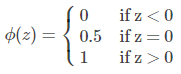但是该分段函数不连续，希望有一个单调可微的函数来让我们使用，于是就找到了sigmoid函数来使用。sigmoid函数的定义如下：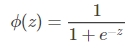二者的关系如左图所示，因为sigmoid函数的取值为[0,1]，可以将其视为类1的后验概率p(y=1|x)，表示测试点x属于类别1 的概率有多大。
此外，我们将sigmoid函数最后计算得到的值大于0.5的分类为1，小于0.5的分类为0：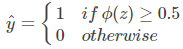问题：为什么使用sigmoid函数做假设？
因为线性回归模型预测的值为实数，而样本标记的分类为（0，1）之间，所以必须要将分类任务的真实标记y与线性回归模型的预测值联系起来，也就是找到一个广义线性模型中的联系函数。
如果选择单位阶跃函数的话，它是不连续的不可微的。而sigmoid函数是连续的，可以将z转化为一共接近0或1的值。
2、逻辑回归的假设
其第一个假设是：假设数据服从伯努利分布
其第二个假设是：假设模型的输出值是样本为正的概率。
所以整个模型可以描述为，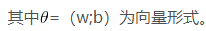3、逻辑回归的代价函数
要根据给定的训练集，将参数w求出来，所以先定义代价函数，使用误差平法和来当作代价函数：

将带入的话，会发现这是一个非凸函数，这就意味着代价函数有着许多的局部最小值，不利于求解。
而最大似然作为逻辑回归模型的损失函数，很容易得到参数的最优解（凸函数）。这就是说选取的标准更容易测量，能够求得最优解。
4、极大似然估计
逻辑回归与极大似然估计的关系：
最大似然估计：是通过已知的结果反推处导致最大结果的参数，而极大似然估计是概率论在统计学中的应用，它提供了一种给定观察数据来评估模型参数的方法，即“模型已确定，参数未确定”，通过若干次实验观察，利用实验的某个参数使得样本出现的概率最大，称为极大似然轨迹。
逻辑回归是一种监督式学习，有标签。即从已知的结果入手，去推导出能够获得最大概率的结果参数，只要能找到这个参数，那么此模型就可以较为准确的预测数据了。
之前提到了可以视为类1的后验概率，所以有：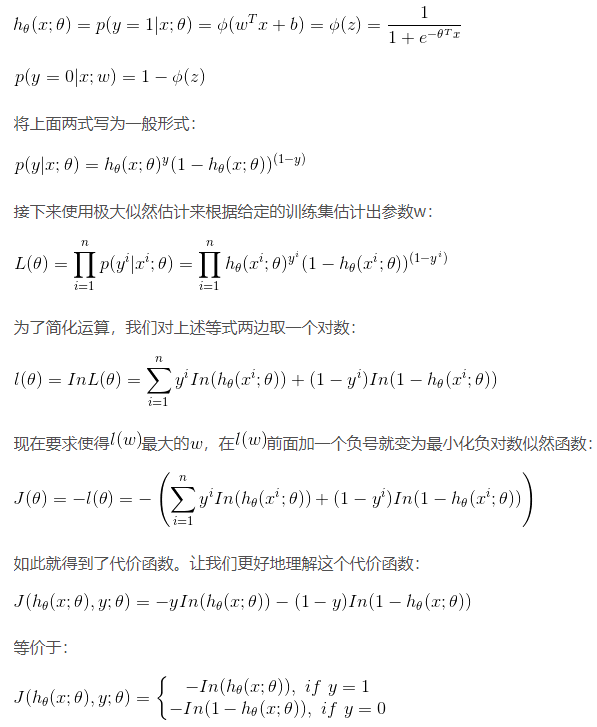可以看出，如果样本的类别为1，估计值越接近1付出的代价越小，反之越大。
同理，如果样本的值为0的话，估计值越接近于0付出的代价越小，反之越大。
5、利用梯度下降发求解参数w
为什么梯度的负方向就是代价函数下降最快的方向？
借助于泰勒展开：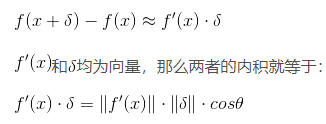当时，也就是在的负方向时，取得最小值，也就是下降的最快方向了。
梯度下降：

为学习率，用来控制步长所以，在使用梯度下降法更新权重时，只要根据下式即可：

（代表第j列特征，代表第j个特征对应的参数）
当样本量极大时，每次权重更新都需要遍历整个数据集，会非常耗时，此时可以采用随机梯度下降方法：

每次只利用1个样本点来更新回归系数，这种方法被称为随机梯度上升法，占用的资源会更少。
6、三种梯度下降方法的比较
优点：可以获得全局最优解，易于并行实现；
缺点：更新参数时需要遍历所有数据，计算量很大，更新慢
2、随机梯度下降SGD：
优点：训练速度快
缺点：准确率下降，得到的并不是全局最优，不利于并行实现。
具体设计思路：更新参数时，只使用一个样本来更新
3、small batch梯度下降：
结合上述两种方法的优点，每次更新参数时只使用一部分样本，减少参数更新的次数，可以达到更加稳定的结果，深度学习中用的很多。
7、逻辑回归的优缺点：
优点：
1.直接对分类可能性进行建模，无需实现假设数据分布，这样就避免了假设分布不准确所带来的问题（周志华.机器学习）
（其实很多机器学习模型本身都是对数据分布有一定的假设的，在这个假设前提之下去进行理论研究有助于我们关注主要矛盾，忽律次要矛盾。但是在工程当中，很多时候我们对数据的分布其实是不了解的，贸然对数据进行假设容易造成模型无法无法拟合真实的分布。） 2、形式简单，模型的课解释好，可以看到不同特征对最后结果的影响。
3、除了得到类别之外，还能得到近似概率预测
缺点：
1、准确率不高，形式较为简单，很难对真实数据进行模拟
2、本身无法筛选特征


展开全文• 原文转自机器学习算法之线性模型 线性方程与非线性方程 线性方程：代数方程如y = 2x + 5,其中任何一个变量都为1次幂，这种方程的图像为一条...线性模型（线性回归；逻辑回归——对数几率回归） ...
• 请阅读《机器学习之矩阵微积分及其性质》和《机器学习之线性回归公式推导》。首先我们还是使用如下的数据： feature_1 feature_2 feature_n value 1 ... 2 ......机器学习 公式推导
• 逻辑回归和其他回归的异同，及其如何选择关于权值的更新函数问题。用最大似然估计来选取costfunction
• 为了知其然还要知其所以然，本篇文章就从最统计学最基础的最大似然估计来推导线性回归和逻辑回归的损失函数。 最大似然估计 通俗地来说，最大似然估计所要达成的目标就是找到一组参数使得表达的概率分布能够在最大...机器学习 算法
• 目录 1.概率模型和非概率模型 1.1 非概率模型 1.1 概率模型 2 频率学派和贝叶斯学派 ...要介绍极大似然估计最大后验估计，就要先从概率模型和非概率模型说起。极大似然估计最大后验估计都.机器学习
• 老师要求我们对回归方程中的回归系数进行极大似然估计回归方程如下： 正常的步骤应该是这样的： 步骤一：写出极大似然函数log(β)，其中的β为(β0,β1,β2)t(β_0, β_1, β_2)^t(β0​,β1​,β2​)t 步骤二：...统计学
• 为了解决在估计条件自回归极差模型...证明了对数正态分布下的拟极大似然估计是局部相合和渐近正态的，并且对数正态分布的厚尾性也较好地解决了异常值问题相对于目前广泛采用的指数似然估计方法，提高了参数估计的效率。
• 背景知识常见回归模型线性回归(linear regression)：y=wTx+b(1) y=\mathbf{w}^T\mathbf{x}+b\tag{1} 但是有时候预测值会逼近y\mathbf{y}的衍生值比如输出标记在指数尺度上变化。对数线性回归(log-linear ...机器学习
• 通俗理解来说，就是利用已知的样本的结果信息，反推最具有可能（最大概率）导致这些样本结果出现的原因，在极大似然估计中，所谓的“原因”指的是模型参数值！换句话说，极大似然估计提供了一种给定观察数据来评估...
• 例如，我们可以使用一个随机森林模型来分类客户是否会取消订阅服务（称为流失建模），或者我们可以用线性模型根据公司的广告支出来预测公司的收入（这是一个线性回归的例子）。每个模型都包含自己的一组参数，这些...贝叶斯估计 参数估计
• ## 极大似然估计详解，写的太好了！

万次阅读 多人点赞 2018-08-18 15:42:08
极大似然估计  以前多次接触过极大似然估计，但一直都不太明白到底什么原理，最近在看贝叶斯分类，对极大似然估计有了新的认识，总结如下：   贝叶斯决策  首先来看贝叶斯分类，我们都知道经典的贝叶斯公式：...python
• 机器学习EM算法以及逻辑回归算法模型参数的求解都用到了最大似然估计，本文讲解其原理。 极大似然估计，通俗理解来说，就是利用已知的样本结果信息，反推最具有可能（最大概率）导致这些样本结果出现的模型参数值！...
• 针对带有协变量误差的部分线性回归模型的估计问题，利用经验似然方法，在一定条件下，证明了所得到的未知参数的极大经验似然估计具有渐近正态性。同时获得了非参数估计的最优收敛速度OP（n-1/3）。
• 极大似然估计 博客转载自：https://blog.csdn.net/raintungl/article/details/78188182 本篇博客主要讲解以下内容： 一、参数估计 二、极大释然估计 1．似然函数 2．极大似然估计（MLE） 3．log似然函数 4．损失...参数估计
• 统计学中，我们经常能听到极大似然估计，或者最大似然估计，它是一种参数估计方法。在机器学习中，逻辑回归就是基于极大似然估计来计算的损失函数。那么，如何直观理解极大似然估计极大似然估计 极大似然估计...
• 02 回归算法 - 线性回归求解 θ（最大似然估计求解）白尔摩斯关注0.2942018.10.06 18:32:53字数 3,014阅读 4,876回顾线性回归的公式：θ是系数，X是特征，h(x) 是预测值。 h(x) = θ0 + θ1x1 + θ2x2 + … + θnxn ...
• 文章目录1、最大似然估计的一般理论2、最大似然估计的性质3、最大似然估计推导逻辑回归的损失函数4、线性回归的损失函数5、小结 1、最大似然估计的一般理论 我们希望可以有些准则可以让我们从不同的模型中得到特定...损失函数
• 回归问题普遍讨论的是多元线性回归，考虑多个特征可以得到更精确的模型，这其中涉及中心极限定理，正态分布，概率密度函数和最大似然估计。 (一)背景——多元线性回归 1. 本质上就是算法（公式）变换为了多元一次...机器学习
• ## 最大似然估计解决线性回归

万次阅读 多人点赞 2017-05-04 17:10:52
我们得到一堆数据（xi,yi）,现在要用线性方程的形式去做回归，线性方程结构为：y=f(x)+e,其中f(x)=w*x，w是我们要进行估计的参数向量，e是噪声，且该噪声服从均值为0高斯分布，即：e~N(0,sigema^2)。 假如说现在有...
• 转自 ...   对原文增加理解，其中: 1. s0, s1式是直接对下式进行求对数得到 2. 综合两种情况：  ...β)=y1βTx̂ −ln(1+eβTx̂ ) ...β) = y1lnp1 + (1-y1)lnp0， 然后将s0, s1代入...机器学习
• 本文以一元线性回归为例，整理线性回归模型参数的估计方法。 样本(x,y)(x,y)(x,y)可由y=β0+β1x+εy=\beta _{0}+\beta _{1}x+\varepsilony=β0​+β1​x+ε 表示，其中，ε\varepsilonε为随机因素引起的噪声， y=...
• 机器学习的损失函数是人为设计的，用于评判模型...其次，既然不同标准并不冲突，那使用最小二乘作为逻辑回归的损失函数当然是可以，那这里为什么不用最小二乘而用最大似然呢？请看一下最小二乘作为损失函数的函数......